Block
Are you looking to get certified in DevOps, SRE and DevSecOps?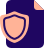DevOps
Get Certified!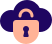SRE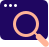DevSecOps
Security is Key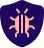Kubernetes
Tomorow's Platform!

# Comparison or Relational Operators in JavaScript.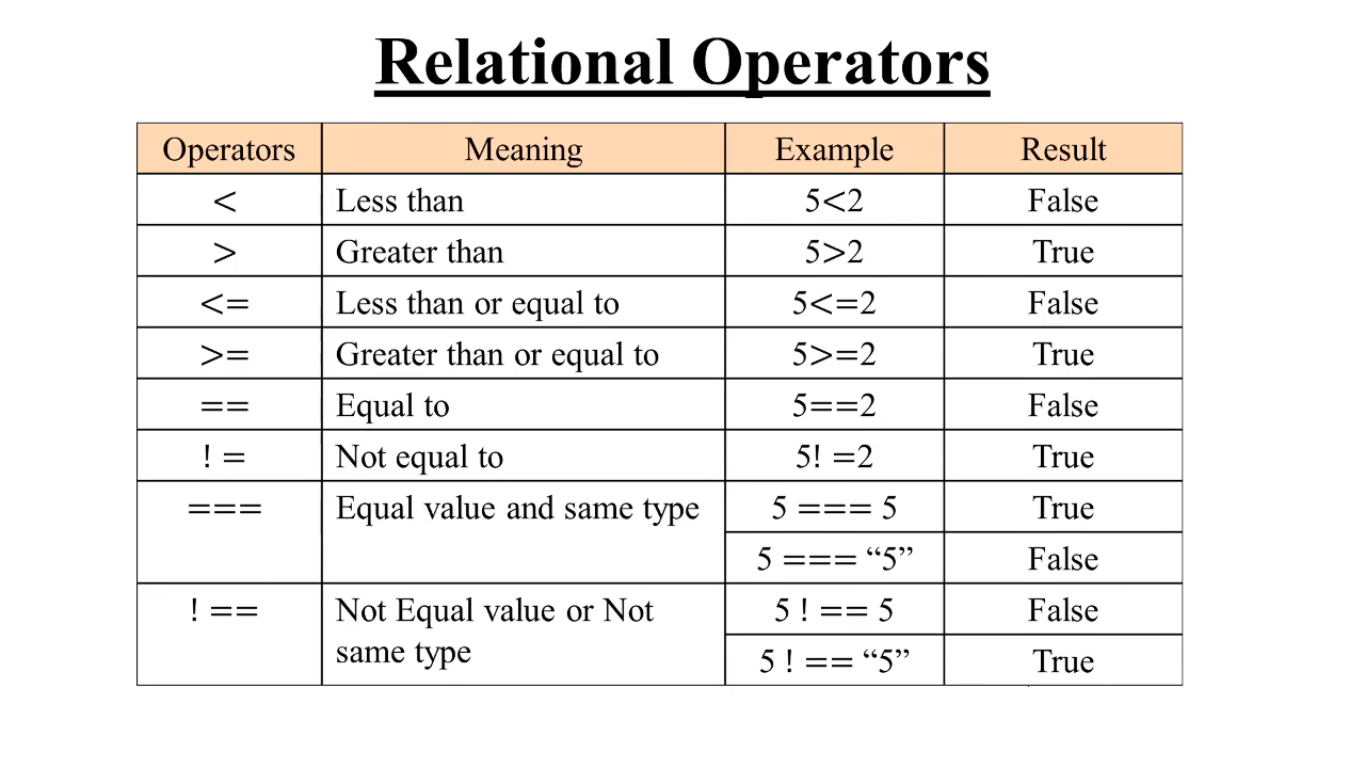1 . Less than ( < ) :- This operation will return TRUE when the expression will be satisfied and FALSE when not satisfied. See the Below Example

var a = 5<2 ;
document.write(a) ;

OUTPUT :- False

2 . Greater than ( > ) :- This operation will return TRUE when the expression will be satisfied and FALSE when not satisfied. See the Below Example

var a = 5>2 ;
document.write(a) ;

OUTPUT :- True

3 . Less than or Equal to ( <= ) :- When 1st element is Less than or Equal to the other element then it returns TRUE otherwise FALSE. See the Below Example

var a = 5<=2 ;
document.write(a) ;

OUTPUT :- False

4 . Greater than or Equal to ( >= ) :- When 1st element is Greater than or Equal to the other element then it returns TRUE otherwise FALSE. See the Below Example

var a = 5>=2 ;
document.write(a) ;

OUTPUT :- True

5 . Equal to ( == ) :- When 1st element is Equal to the other element then it returns TRUE otherwise FALSE. See the Below Example

var a = 5==2 ;
document.write(a) ;

OUTPUT :- False

6 . Not Equal to ( != ) :- When 1st element is Not Equal to the other element then it returns TRUE otherwise FALSE. See the Below Example

var a = 5!=2 ;
document.write(a) ;

OUTPUT :- True

7 . Equal Value and Same Type ( === ) :- When 1st element is Equal to and of the Same Type to the other element then it returns TRUE otherwise FALSE. See the Below Example

var a = 5===5 ;
document.write(a) ;

OUTPUT :- True
Note :- in the above example the elements are of equal value and same type, so it returns TRUE.
————————————————————————————————–
var a = 5===”5″ ;
document.write(a) ;

OUTPUT :- FALSE
Note :- in the above example the elements are of equal value but not of same type, so it returns TRUE.

8 . Not Equal Value or Not Same Type ( === ) :- When 1st element is Not Equal to or Not Same Type to the other element then it returns TRUE otherwise FALSE. See the Below Example

var a = 5!==5 ;
document.write(a) ;

OUTPUT :- FALSE
Note :- in the above example the elements are of equal value or the same type, therefore it can’t satisfy the expression and returns FALSE.
————————————————————————————————–
var a = 5!==”5″ ;
document.write(a) ;

OUTPUT :- TRUE
Note :- in the above example the elements are of equal value but not of same type, therefore it satisfies the expression and returns TRUE.

## Video Reference